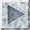# Funthematics

### Fun with Mathematics

•Fun mathematics (by Peter R. Johansson)
some nice pictures produced by mathematical software
•Pure Mathematics for Fun (by Mike Alder)
several nice fractals and 4-dimensional animated projections
•Mathematical theory of big game hunting (offered by Frank Wikström)
article first published in 1938 in American Mathematical Monthly by H. Petard, of Princeton, NJ
•Satan and the Slacker (by the University of Warwick Mathematics Society)
Is nothing the same as infinity? Is Martin Gardner the Devil? Read on...
•This is MEGA Mathematics! (by the Los Alamos National Laboratory)
Have fun with Hotel Infinity and other strange entities...
•WELCOME TO MY PAGE OF PI! (by Olov "Olle" Windelius)
everything you did and didn't want to know about the transcendent number pi - highlight: in Indiana, USA, 1897, there was almost a law that statedpi = 3...
•Bamdad's Math Comics Page (by Bamdad)
great collection of math related comics and cartoons
•Fun With Mathematics! (by Harry J. Smith)
many entertaining math subjects

### Mathematical Games

•...proving that mathematics can be fun! (by Michael Curl Internet Publishing)
mathematical brain games (find the biggest prime, crossfigures (= numerical crosswords)) and visual testlab (Explore the wallpaper groups!, demonstration of the normal distribution curve), links to sites with mathematical puzzles and mathematical recreations, books on math & logic puzzles, mathematical recreations and fractals

### Mathematical Jokes

•Fun Stuff (by the University of Warwick Mathematics Society)
jokes about mathematics and mathematicians, categorized (and some other stuff...:-)
•A few mathematical jokes (by Frank Wikström)
nicely edited
•Science Jokes Archive (by Paul E. Mayer)
a huge archive of science related jokes (including a great math section)

Back to the Top